## FRP Design Software - Flexural Strengthening Of RC Slabs With CF

FRP Calculations of Slab With CFRP Plate

FRP Design Software - Flexural Strengthening Of RC Slabs With CFRP Plate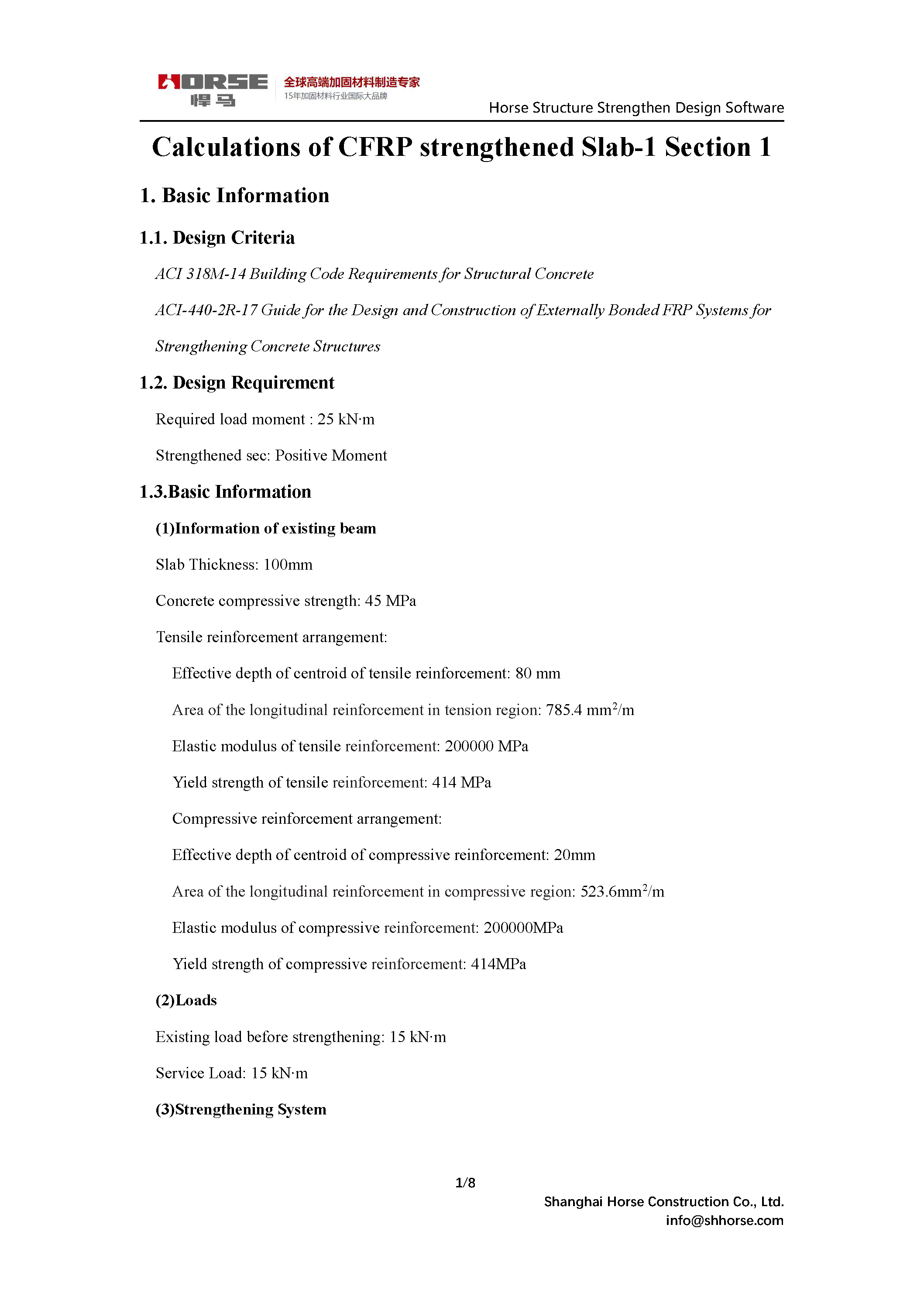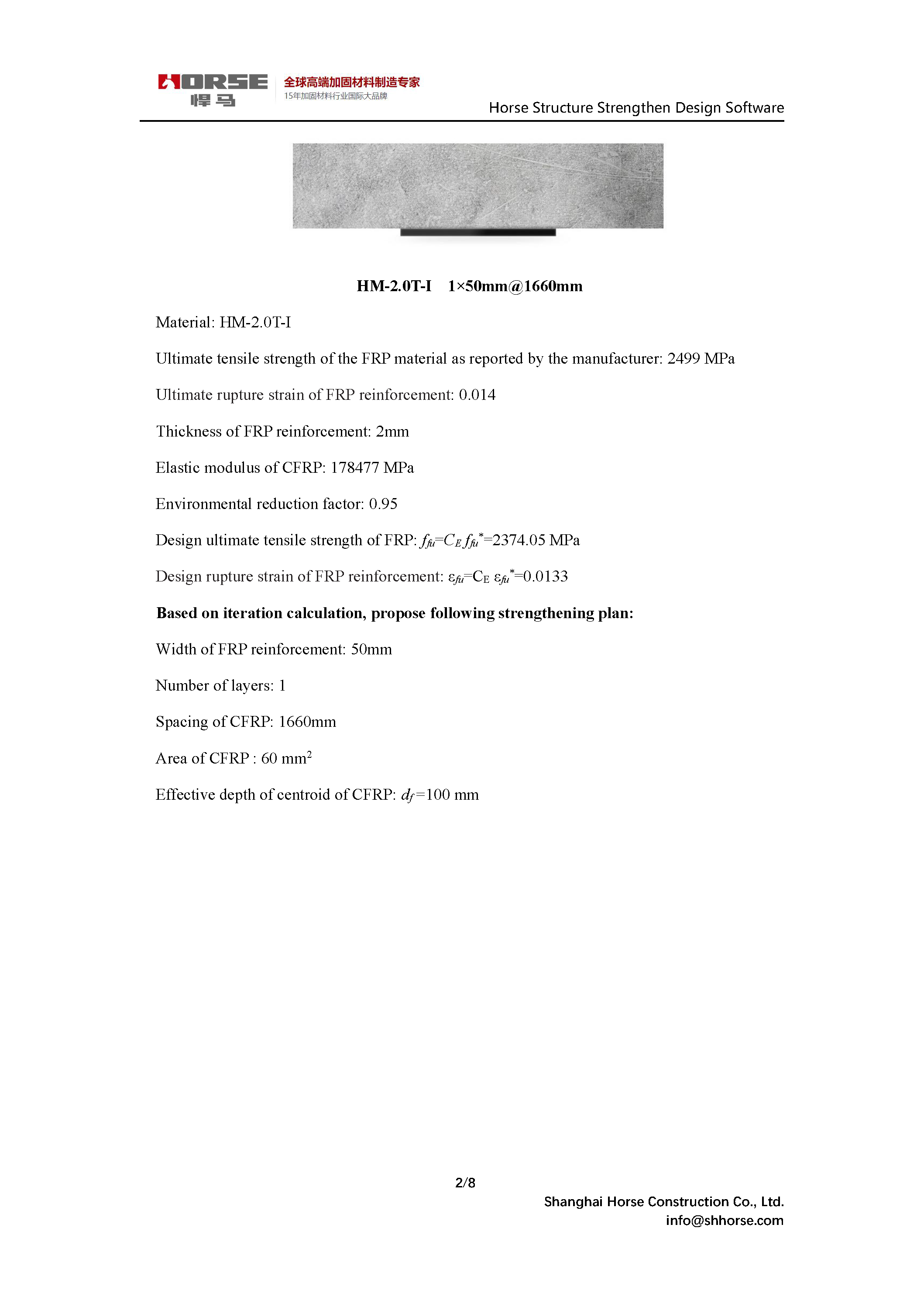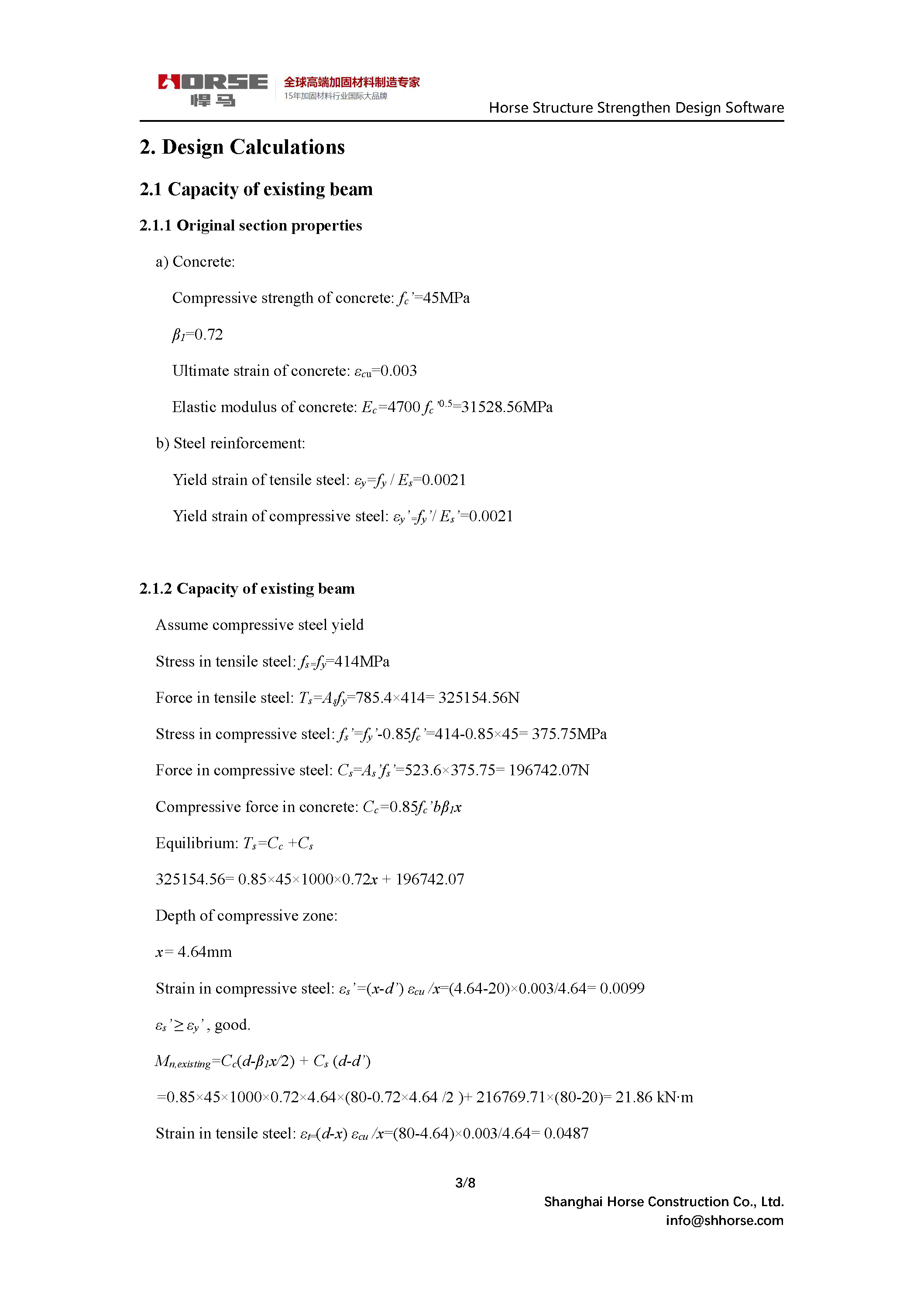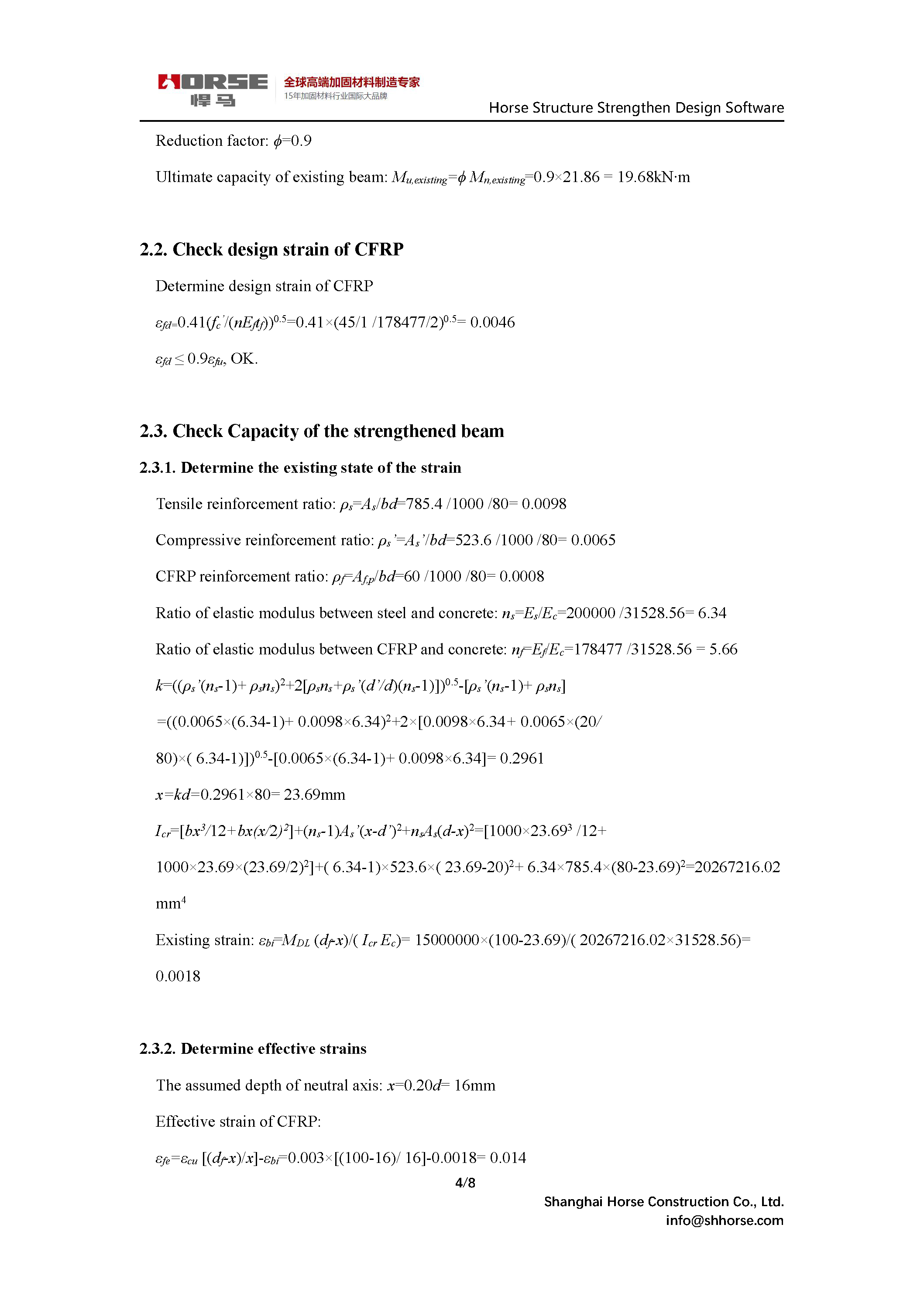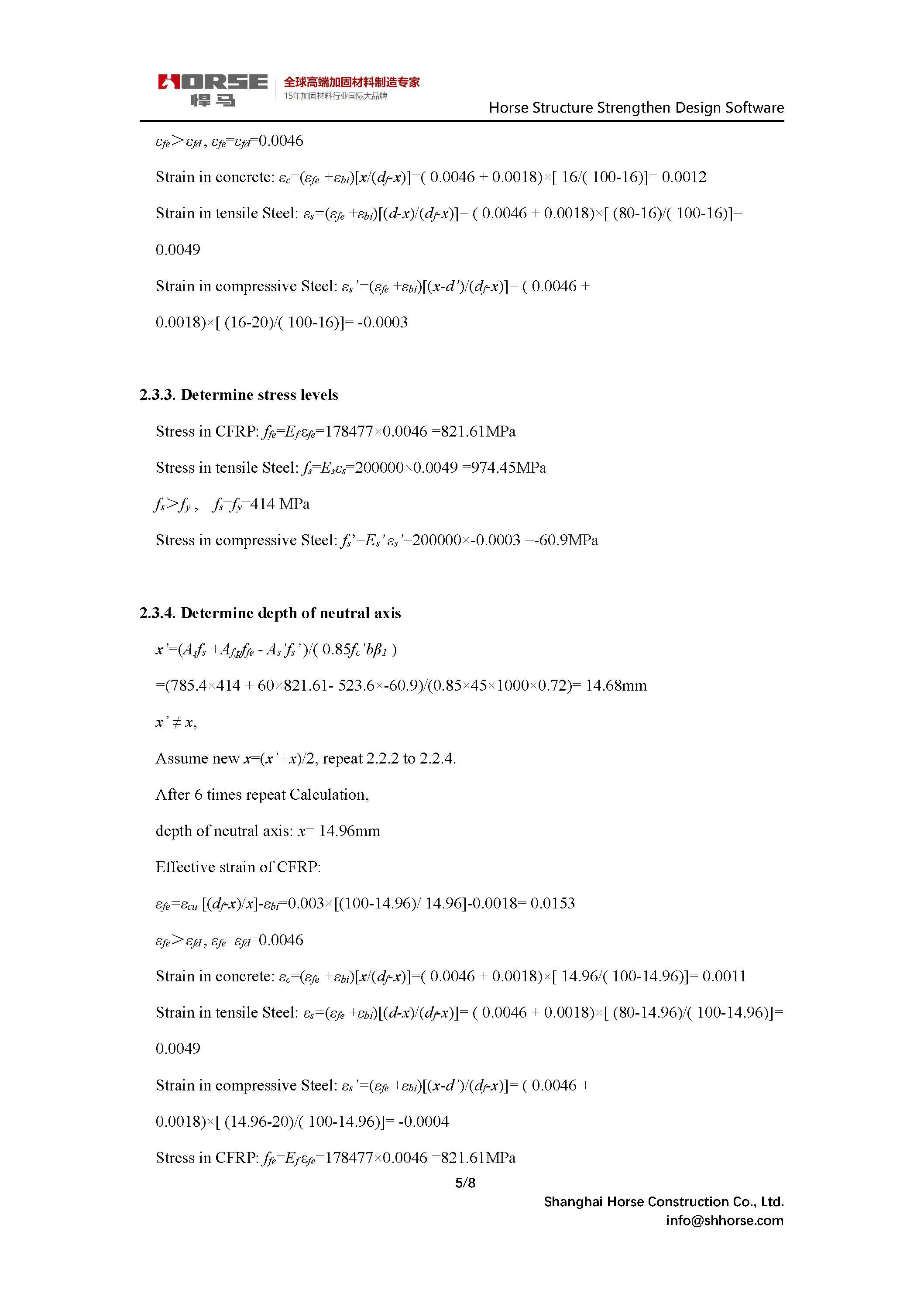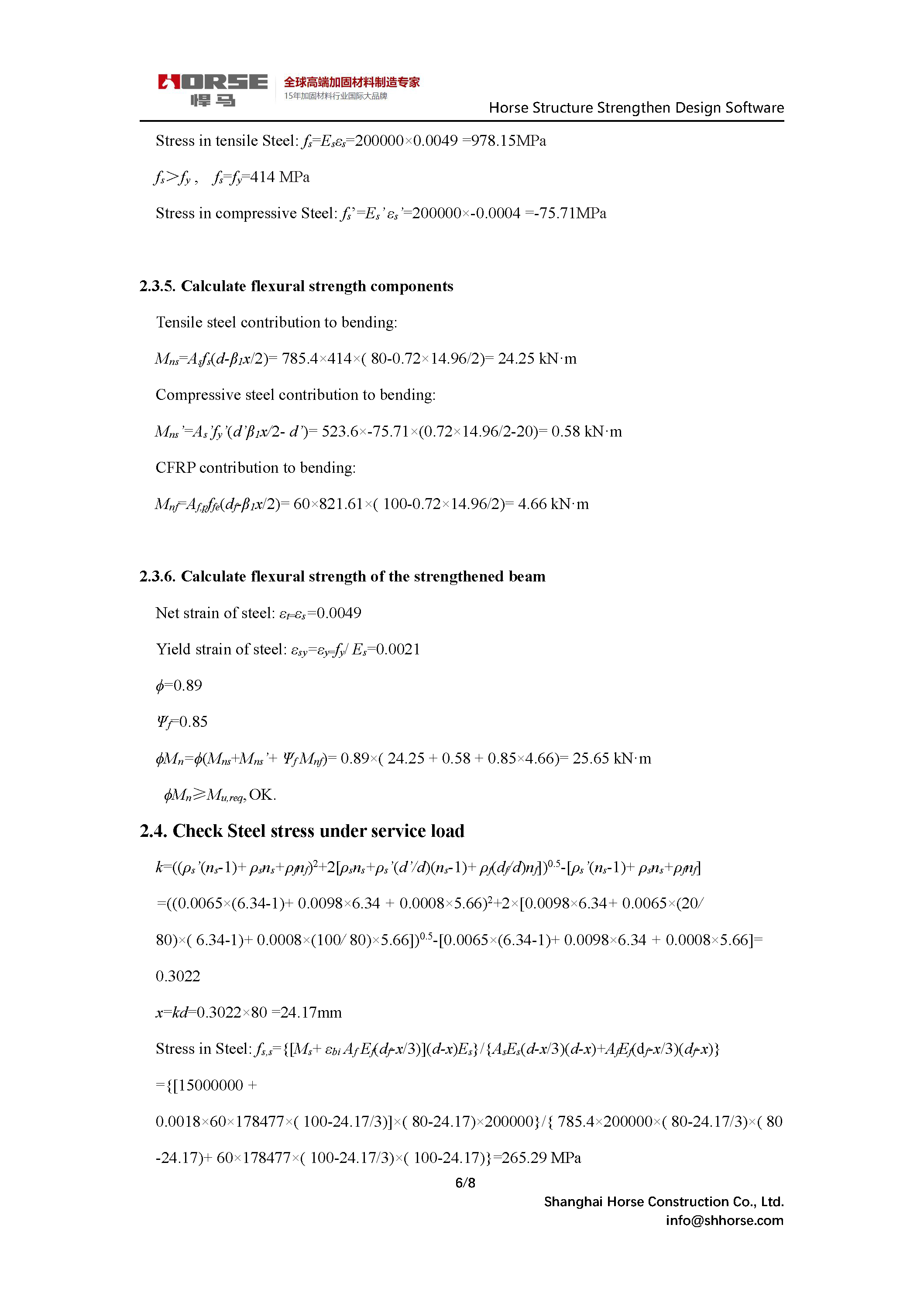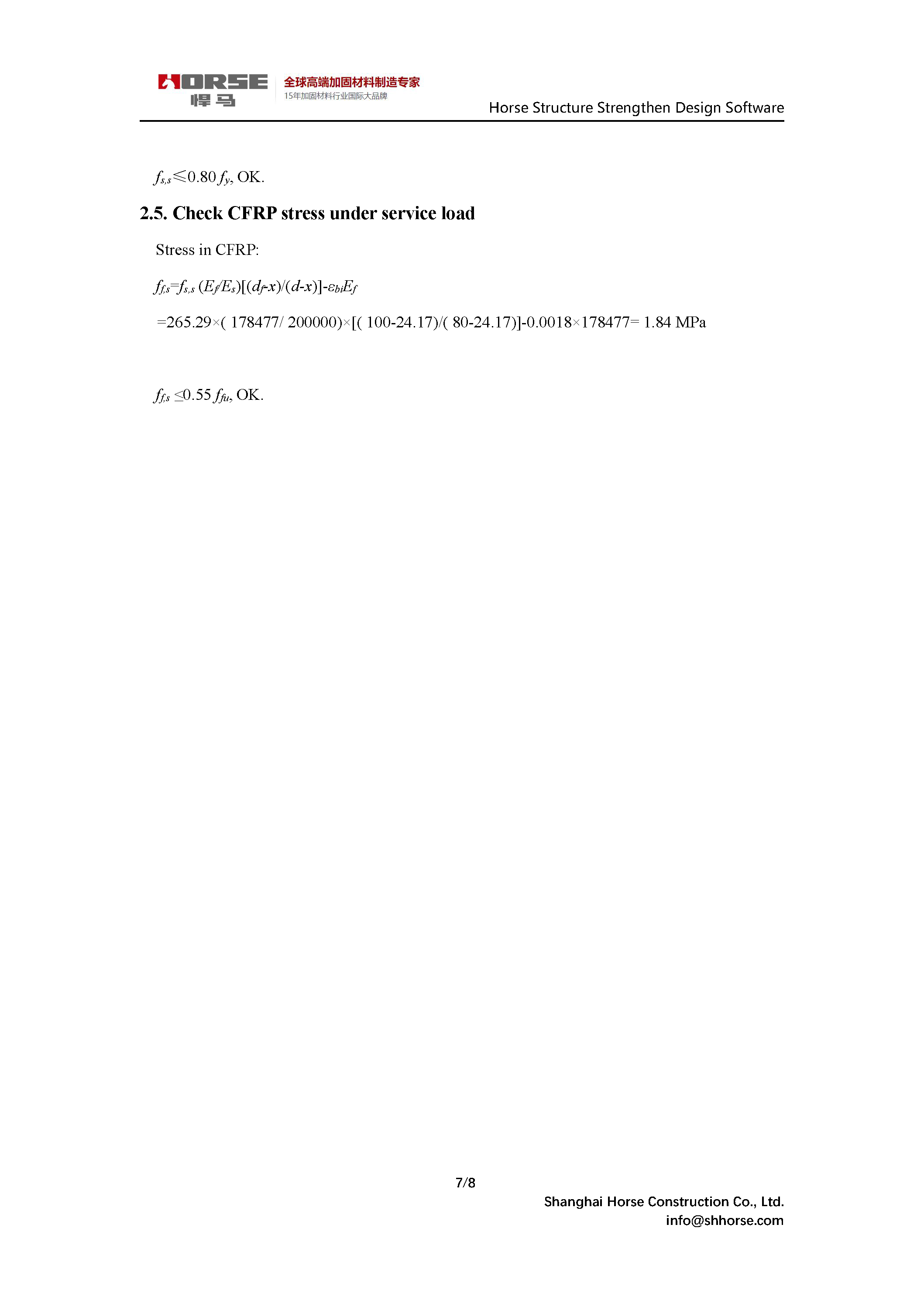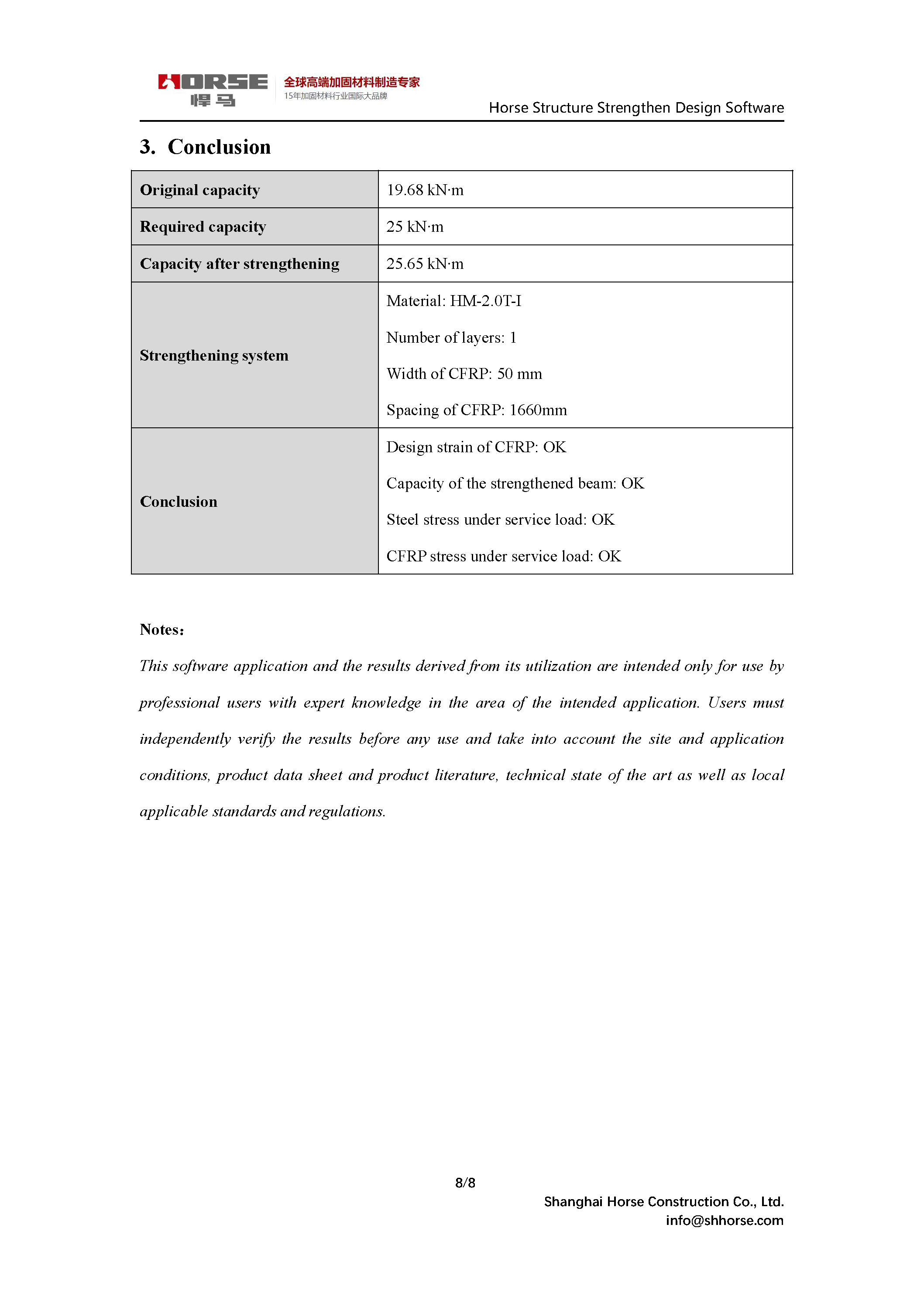1. Basic Information

1.1. Design Criteria

ACI 318M-14 Building Code Requirements for Structural Concrete

ACI-440-2R-17 Guide for the Design and Construction of Externally Bonded FRP Systems for Strengthening Concrete Structures

1.2. Design Requirement

Required load moment : 25 kNm

Strengthened sec: Positive Moment

1.3.Basic Information

(1)Information of existing beam

Slab Thickness: 100mm

Concrete compressive strength: 45 MPa

Tensile reinforcement arrangement:

Effective depth of centroid of tensile reinforcement: 80 mm

Area of the longitudinal reinforcement in tension region: 785.4 mm2/m

Elastic modulus of tensile reinforcement: 200000 MPa

Yield strength of tensile reinforcement: 414 MPa

Compressive reinforcement arrangement:

Effective depth of centroid of compressive reinforcement: 20mm

Area of the longitudinal reinforcement in compressive region: 523.6mm2/m

Elastic modulus of compressive reinforcement: 200000MPa

Yield strength of compressive reinforcement: 414MPa

Existing load before strengthening: 15 kNm

(3)Strengthening System

Material: HM-2.0T-I

Ultimate tensile strength of the FRP material as reported by the manufacturer: 2499 MPa

Ultimate rupture strain of FRP reinforcement: 0.014

Thickness of FRP reinforcement: 2mm

Elastic modulus of CFRP: 178477 MPa

Environmental reduction factor: 0.95

Design ultimate tensile strength of FRP: ffu=CE ffu*=2374.05 MPa

Design rupture strain of FRP reinforcement: εfu=CE εfu*=0.0133

Based on iteration calculation, propose following strengthening plan:

Width of FRP reinforcement: 50mm

Number of layers: 1

Spacing of CFRP: 1660mm

Area of CFRP : 60 mm2

Effective depth of centroid of CFRP: df =100 mm

2. Design Calculations

2.1 Capacity of existing beam

2.1.1 Original section properties

a) Concrete:

Compressive strength of concrete: fc=45MPa

β1=0.72

Ultimate strain of concrete: εcu=0.003

Elastic modulus of concrete: Ec=4700 fc0.5=31528.56MPa

b) Steel reinforcement:

Yield strain of tensile steel: εy=fy / Es=0.0021

Yield strain of compressive steel: εy=fy/ Es=0.0021

2.1.2 Capacity of existing beam

Assume compressive steel yield

Stress in tensile steel: fs=fy=414MPa

Force in tensile steel: Ts=Asfy=785.4×414= 325154.56N

Stress in compressive steel: fs=fy-0.85fc=414-0.85×45= 375.75MPa

Force in compressive steel: Cs=Asfs=523.6×375.75= 196742.07N

Compressive force in concrete: Cc=0.85fc’bβ1x

Equilibrium: Ts=Cc +Cs

325154.56= 0.85×45×1000×0.72x + 196742.07

Depth of compressive zone:

x= 4.64mm

Strain in compressive steel: εs=(x-d’) εcu /x=(4.64-20)×0.003/4.64= 0.0099

εs εy , good.

Mn,existing=Cc(d-β1x/2) + Cs (d-d’)

=0.85×45×1000×0.72×4.64×(80-0.72×4.64 /2 )+ 216769.71×(80-20)= 21.86 kNm

Strain in tensile steel: εt=(d-x) εcu /x=(80-4.64)×0.003/4.64= 0.0487

Reduction factor: ϕ=0.9

Ultimate capacity of existing beam: Mu,existing=ϕ Mn,existing=0.9×21.86 = 19.68kNm

2.2. Check design strain of CFRP

Determine design strain of CFRP

εfd=0.41(fc/(nEftf))0.5=0.41×(45/1 /178477/2)0.5= 0.0046

εfd 0.9εfu, OK.

# Horse Construction Recommended Products

You can find anything here you are in need of, have a trust trying on these products, you will find the big difference after that.## HM-1.2T Unidirectional Carbon Fiber Strip

High strength carbon fiber reinforced polymer (CFRP) strip / laminate / plate for structural strengthening and concrete repair

See more >